# Flg. 4-51 Problem 91.A projectile is launched with an initial speed of 30 m/s at anangle of 60° above the horizontal. What are the (a) magnitude and(b) angle of its velocity 2.0 s after launch, and (c) is the angle aboveor below the horizontal? What are the (d) magnitude and (e) angleof its velocity 5.0 s after launch, and (f) is the angle above or below105the horizontal?

Question
2 views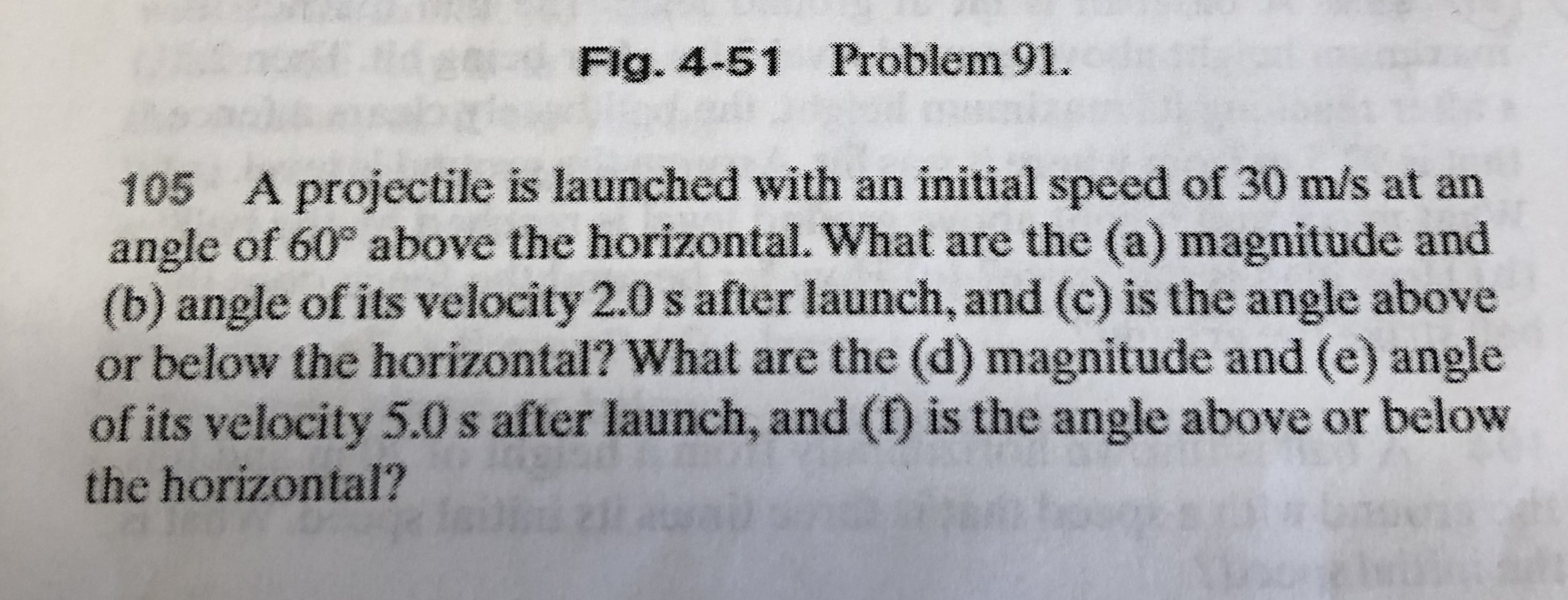help_outlineImage TranscriptioncloseFlg. 4-51 Problem 91. A projectile is launched with an initial speed of 30 m/s at an angle of 60° above the horizontal. What are the (a) magnitude and (b) angle of its velocity 2.0 s after launch, and (c) is the angle above or below the horizontal? What are the (d) magnitude and (e) angle of its velocity 5.0 s after launch, and (f) is the angle above or below 105 the horizontal? fullscreen
check_circle

Step 1

Given information:

Initial velocity of the projectile (u) = 30 m/s

The angle at which it is launched (θ) = 600

Step 2

We can write the components of the velocity as follows:

x-component (ux) = ucos (60) = 15 m/s

y-component (uy) = usin (60) = 25.93 m/s

We know that in projectile motion the x-component of the velocity remains un effected by the gravitational force. But the y-component of the velocity keeps on changing. The y-component of velocity in the varies as follows: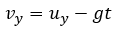Therefore, the y-component of the velocity in after 2 s is given by: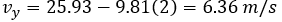Step 3

The magnitude of the velocity after 2 s is given by: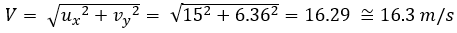The angle of velocity with the horizontal is given by: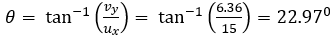Therefore, the velocity vector makes an angle of 22.970 with the horizontal.

Since the angle is +ve we can say that the angle is made above the horizontal.

...

### Want to see the full answer?

See Solution

#### Want to see this answer and more?

Solutions are written by subject experts who are available 24/7. Questions are typically answered within 1 hour.*

See Solution
*Response times may vary by subject and question.
Tagged in